# Difference between revisions of "Levi-Civita connection"

This lives as an element of: the space of all linear connections, which in turn sits inside the space of all$\R$-bilinear maps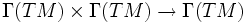$\Gamma(TM) \times \Gamma(TM) \to \Gamma(TM)$

## Definition

### Given data

A Riemannian manifold$(M,g)$ (here,$M$ is a differential manifold and$g$ is the additional structure of a Riemannian metric on it).

More generally, we can also look at a pseudo-Riemannian manifold, or a manifold with a pseudo-Riemannian metric: a smoothly varying nondegenerate (not necessarily positive definite) symmetric bilinear form$g$ in each tangent space.

### Definition part

A Levi-Civita connection on$(M,g)$ is a linear connection$\nabla$ on$M$ satisfying the following two conditions:

• The connection is metric, viz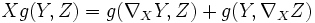$X g (Y,Z) = g (\nabla_X Y, Z) + g(Y, \nabla_X Z)$
• The connection is torsion-free, viz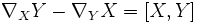$\nabla_X Y - \nabla_Y X = [X,Y]$.

### Definition by formula

The formula for the Levi-Civita connection requires us to use the fact that$g$ is nondegenerate. So, instead of directly specifying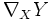$\nabla_XY$ for vector fields$X$ and$Y$, the formula specifies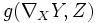$g(\nabla_XY,Z)$ for$X,Y,Z$ vector fields, as follows: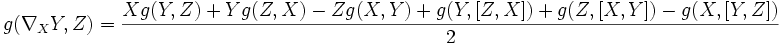$g(\nabla_XY,Z) = \frac{Xg(Y,Z) + Yg(Z,X) - Zg(X,Y) + g(Y,[Z,X]) + g(Z,[X,Y]) - g(X,[Y,Z])}{2}$.

The term Levi-Civita connection is sometimes also used for the connection induced on any tensor product involving the tangent and cotangent bundle, using the rule for tensor product of connections and the dual connection.

## Facts

### The Levi-Civita connection is unique

Further information: Levi-Civita connection exists and is unique

The proof combines the metric condition, the torsion-free condition, and the nondegeneracy of$g$.

Note that the nondegeneracy of$g$ is very important, otherwise knowing the value of the inner product for any three vectors may not necessarily help us in computing the value of$\nabla_XY$

### Christoffel symbols

Further information: Christoffel symbols of a connection

The Levi-Civita connection on a manifold$M$ is a map$\nabla: \Gamma(TM) \times \Gamma(TM) \to \Gamma(TM)$. This means that at any point$p \in M$, it gives a map$T_p(M) \times \Gamma(TM) \to \Gamma(TM)$, which roughly differentiates one tangent vector along another.

Let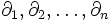$\partial_1, \partial_2, \ldots, \partial_n$ form a basis for the tangent space$TM$. Then, the Christoffel symbol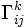$\Gamma_{ij}^k$ is the component along$e_k$ of the vector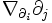$\nabla_{\partial_i}\partial_j$.

The Christoffel symbols thus give an explicit description of the Levi-Civita connection. Namely, the Levi-Civita connection can be expressed using the Christoffel symbols.